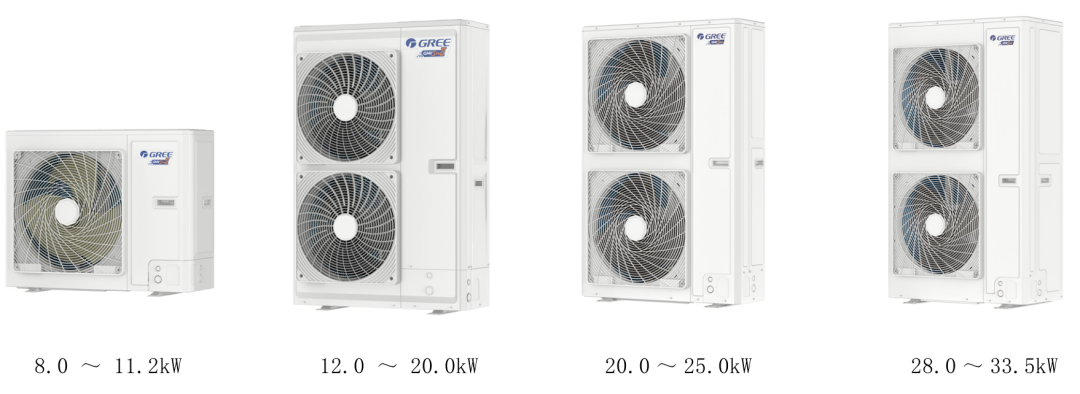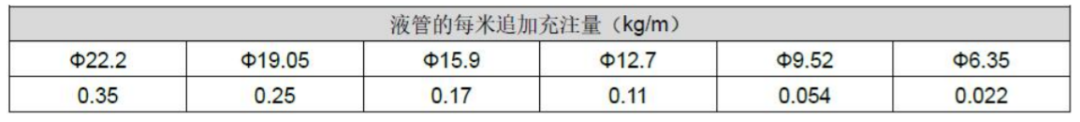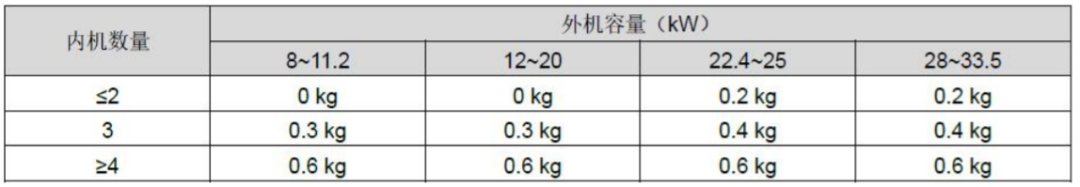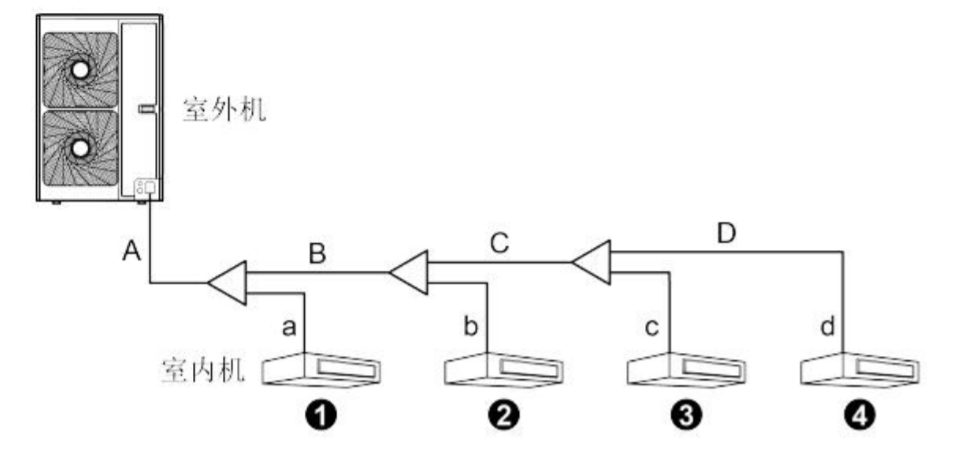### 格力新装StarⅡ家庭中央空调为什么要补加制冷剂？（1） 追加制冷剂量 R=配管制冷剂追加量 A+外机制冷剂追加量 B

（2） 配管制冷剂追加量 A 计算方法如下：配管制冷剂追加量 A=Σ液管长度×每米液管制冷剂追加量。（3） 外机制冷剂追加量 B 计算方法如下：计算示例 以 GMV-H160WL/H2 为例：Φ9.52: A+B+C+a=15+8+7+3=33m

Φ6.35: D+b+c+d=5+3+2+1=11m